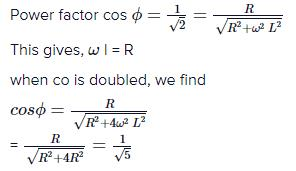Courses

# Test: Power In an AC Circuit

## 10 Questions MCQ Test Physics For JEE | Test: Power In an AC Circuit

Description
This mock test of Test: Power In an AC Circuit for JEE helps you for every JEE entrance exam. This contains 10 Multiple Choice Questions for JEE Test: Power In an AC Circuit (mcq) to study with solutions a complete question bank. The solved questions answers in this Test: Power In an AC Circuit quiz give you a good mix of easy questions and tough questions. JEE students definitely take this Test: Power In an AC Circuit exercise for a better result in the exam. You can find other Test: Power In an AC Circuit extra questions, long questions & short questions for JEE on EduRev as well by searching above.
QUESTION: 1

### Find the total voltage applied in a series RLC circuit when i=3mA, VL=30V, VC=18V and R=1000 ohms.

Solution:

Explanation: Total voltage= VR+VL+VC.
VR=1000x3x10-3=3V.
Therefore, total voltage = 30+18+3=51V.

QUESTION: 2

### The power factor of an RL circuit 1/root2. If the frequency of a.c. is doubled, what will be the power factor?​

Solution:QUESTION: 3

### When an emf E = 7cos wt is applied across a circuit, the current is I = 5coswt. What is the power factor for the circuit?

Solution:

Since E and I are in the same phase.
Therefore, phase difference will be 0 and since power factor= cosx (where x= phase difference) and x =0
therefore, cos x or power factor will be =1

QUESTION: 4

What is value of power factor if Ø = 90°​

Solution:

P=Irms Vrms cos ϕ
Where, ϕ=90
cosϕ=0
therefore,P=0
hence the correct answer is option A.

QUESTION: 5

Power in an ac circuit is equal to

Solution:
QUESTION: 6

If the instantaneous current in a circuit is given by i = 2 cos (ωt - φ) A, the rms value of the current is

Solution:

i=2cost
=Im​cost
So, Im​=2amp
IRMS​=Im​​/√​2​
=2/√​2​
=2​amp

QUESTION: 7

Find the true power given apparent power = 10 W and power factor = 0.5​

Solution:

The formula of true power is,
True power=Apparent power x power factor
So, true power=10 x 0.5
True power=5W

QUESTION: 8

What is the average power/cycle in a capacitor?​

Solution:

The average power consumed/cycle in an ideal capacitor is 0.
The average power consumed in an ideal capacitor is given as based on the instantaneous power which is supplied to the capacitor:
pc = iv= (im cos ωt)(vm sin ωt)
pc = imvm (cos ωt sin ωt)
pc=(imvm/2)sin2ωt
We know that sin ωt = 0
Therefore, average power = 0

QUESTION: 9

What is phase angle given R = 10, Z = 20​

Solution:

Cos x (power factor) = R/Z
= 10/20
=1/2
x= 60

QUESTION: 10

In a circuit, the reactance X and resistance R are equal. What is the power factor?​

Solution:

Given that,
Reactance (X) =Resistance (R)
tanϕ = X/R​
So, we have ϕ = π/4​
Power factor = cosϕ = ​1​/√2